Filters - Page 22d multisection filter circuits between the output of the rectifier and the load. There are four basic types of filter circuits: ">Custom SearchFILTERS While the output of a rectifier is a pulsating dc, most electronic circuits require a substantially pure dc for proper operation. This type of output may be provided by placing single or multisection filter circuits between the output of the rectifier and the load. There are four basic types of filter circuits: Simple capacitor filter LC choke-input filter LC capacitor-input filter (pi-type) RC capacitor-input filter (pi-type) We will cover the function of each of these filters in detail later in this chapter. Filtering is done by using various combinations of capacitors, inductors, and resistors. Inductors are used as series impedances to oppose the change in flow of alternating (pulsating dc) current. Capacitors are used as shunt elements to bypass the alternating components of the signal around the load (to ground). Resistors are used in place of inductors in low current applications. Let's briefly review the properties of a capacitor. First, a capacitor opposes any change in voltage. The opposition to a change in voltage is called capacitive reactance (XC) and is measured in ohms. The capacitive reactance is determined by the frequency (f) of the applied voltage and capacitance (C) of the capacitor.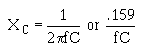From the formula, you can see that if frequency or capacitance is increased, the XC will decrease. Since filter capacitors are placed in parallel with the load, a low XC will provide better filtering than a high XC. This is done by providing a better shunting effect of the ac around the load, as shown in figure 3-15. Figure 3-15. - Capacitor filter. Fast charge time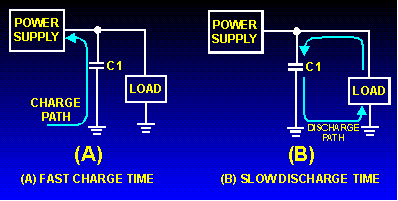To obtain a steady dc output, the capacitor must charge almost instantaneously to the value of applied voltage. Once charged, the capacitor must retain the charge as long as possible. The capacitor must have a short charge time constant (view A) and a long discharge time constant (view B). This can be done by keeping the internal resistance of the power supply as small as possible (fast charge time) and the resistance of the load as large as possible (slow discharge time). From your earlier studies in basic electricity, you may remember that one capacitor time constant is defined as the time it takes a capacitor to charge to 63.2 percent of the applied voltage or to discharge to 36.8 percent of its total charge. This can be expressed by the following equation: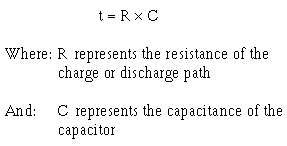You should also recall that a capacitor is considered fully charged after five RC time constants. Referring to figure 3-16, you should see that to obtain a steady dc output voltage, the capacitor should charge rapidly and discharge as slowly as possible. Figure 3-16. - RC time constant chart.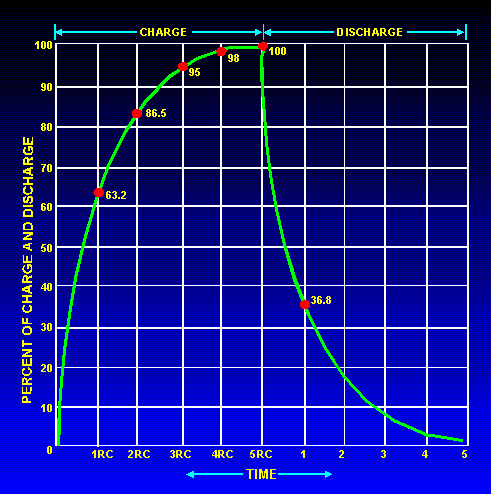In filter circuits the capacitor is the common element to both the charge and discharge paths. Therefore, to obtain the longest possible discharge time, you want the capacitor to be as large as possible. Another way to look at this is: The capacitor acts as a short circuit around the load (as far as the ac component is concerned), and since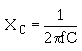the larger the value of the capacitor (C), the smaller the opposition (XC) or resistance to ac. Now let's look at inductors and their application in filter circuits. Remember, AN INDUCTOR OPPOSES ANY CHANGE IN CURRENT. In case you have forgotten, a change in current through an inductor produces a changing electromagnetic field. The changing field, in turn, cuts the windings of the wire in the inductor and thereby produces a counterelectromotive force (cemf). It is the cemf that opposes the change in circuit current. Opposition to a change in current at a given frequency is called inductive reactance (XL) and is measured in ohms. The inductive reactance (XL) of an inductor is determined by the applied frequency and the inductance of the inductor. Mathematically, XL = 2pfL From the preceding formula, you know that if either frequency or inductance is increased, the XL will increase. Since inductors are placed in series with the load (fig. 3-17), the larger the XL, the larger the ac voltage developed across the inductor and the smaller the ac voltage developed across the load. Figure 3-17. - Voltage drops in an inductive filter.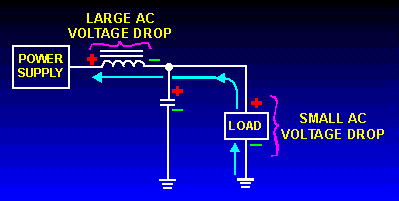Now back to our circuit. As illustrated in figure 3-18, when the current starts to flow through the coil, an expanding magnetic field builds up around the inductor. This magnetic field around the coil develops the cemf that opposes the change in current. When the rectifier current decreases as shown in figure 3-19, the magnetic field collapses and again cuts the turns (windings) of wire, thus inducing current into the coil. This additional current adds to the rectifier current and attempts to keep it at its original level. Figure 3-18. - Inductive filter (expanding field).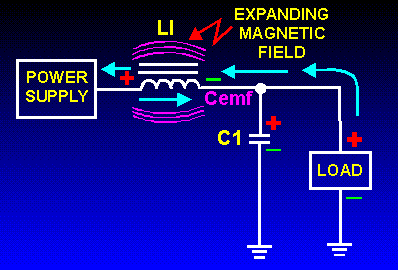Figure 3-19. - Inductive filter (collapsing field).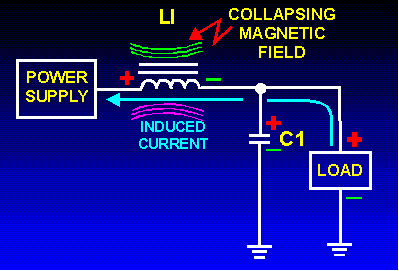Now that you have learned how the components in the filter circuits react to current flow from the rectifier, let's discuss the different types of filter circuits in use today. Q.16 If you increase the value of the capacitor will the XC increase or decrease? Why?Integrated Publishing, Inc. - A (SDVOSB) Service Disabled Veteran Owned Small Business